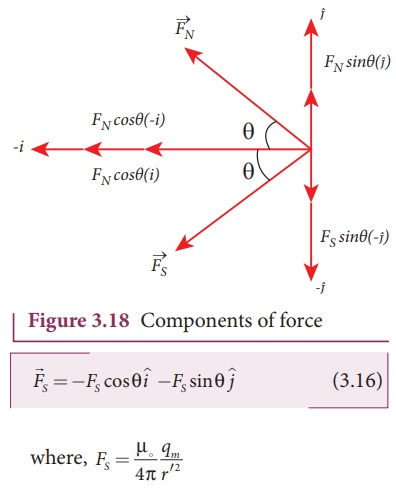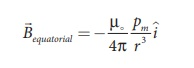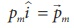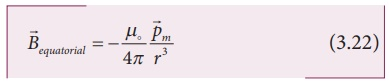Home | | Physics 12th Std | Coulomb’s Inverse Square Law of Magnetism

# Coulomb’s Inverse Square Law of Magnetism

When the north pole of magnet A and the north pole of magnet B or the south pole of magnet A and the south pole of magnet B are brought closer, they repel each other.

COULOMB’S INVERSE SQUARE LAW OF MAGNETISM

Consider two bar magnets A and B as shown in Figure 3.14.

When the north pole of magnet A and the north pole of magnet B or the south pole of magnet A and the south pole of magnet B are brought closer, they repel each other. On the other hand, when the north pole of magnet A and the south pole of magnet B or the south pole of magnet A and the north pole of magnet B are brought closer, their poles attract each other. This looks similar to Coulomb’s law for static charges studiedin Unit I (opposite charges attract and like charges repel each other). So analogous to Coulomb’s law in electrostatics, (Refer unit we can state Coulomb’s law for magnetism (Figure 3.15) as follows:The force of attraction or repulsion between two magnetic poles is directly proportional to the product of their pole strengths and inversely proportional to the square of the distance between them.

Mathematically, we can writewhere mA and mB are pole strengths of two poles and r is the distance between two magnetic poles.where k is a proportionality constant whose value depends on the surrounding medium. In S.I. unit, the value of k for free space iswhere μo is the absolute permeability of free space (air or vacuum).

EXAMPLE 3.5

The repulsive force between two magnetic poles in air is 9 x 10-3 N. If the two poles are equal in strength and are separated by a distance of 10 cm, calculate the pole strength of each pole.

Solution:

The force between two poles are given byThe magnitude of the force isGiven :  F = 9 x 10-3N, r = 10 cm = 10 x 10-2 m

Therefore,## 1. Magnetic field at apoint along the axial line of the magnetic dipole (bar magnet)

Consider a bar magnet NS as shown in Figure 3.16. Let N be the North Pole and S be the south pole of the bar magnet, each of pole strength qm and separated by a distance of 2l. The magnetic field at a point C (lies along the axis of the magnet) at a distance from the geometrical center O of the bar magnet can be computed by keeping unit north pole (qmc = 1 A m) at C. The force experienced by the unit north pole at Cdue to pole strength can be computed using Coulomb’s law of magnetism as follows:

The force of repulsion between north pole of the bar magnet and unit north pole at point C (in free space) iswhere r – l is the distance between north pole of the bar magnet and unit north pole at C.

The force of attraction between South Pole of the bar magnet and unit North Pole at point C (in free space) iswhere r + l is the distance between south pole of the bar magnet and unit north pole at C.From equation (3.9) and (3.10), the net force at point C is. From definition, this net force is the magnetic field due to magnetic dipole at a point C (=)Since, magnitude of magnetic dipole moment isthe magnetic field at a point C equation (3.11) can be written asIf the distance between two poles in a bar magnet are small (looks like short magnet) compared to the distance between geometrical centre O of bar magnet and the location of point C i.e., r >>l then,Therefore,  using  equation  (3.13)  in equation (3.12), we get## 2. Magnetic field at apoint along the equatorial line due to a magnetic dipole (bar magnet)

Consider a bar magnet NS as shown in Figure 3.17. Let N be the north pole and S be the south pole of the bar magnet, each with pole strength q m and separated by a distance of 2l. The magnetic field at a point C (lies along the equatorial line) at a distance r from the geometrical center O of the bar magnet can be computed by keeping unit north pole (qmC = 1 A m) at C. The force experienced by the unit north pole at C due to pole strength N-S can be computed using Coulomb’s law of magnetism as follows:The force of repulsion between North Pole of the bar magnet and unit north pole at point C (in free space) isThe force of attraction (in free space) between south pole of the bar magnet and unit north pole at point C is (Figure 3.18) isFrom  equation  (3.15)  and  equation (3.16), the net force at point C is. This net force is equal to the magnetic field at the point C.In a right angle triangle NOC as shown in the Figure 3.17Substituting equation (3.18) in equation (3.17) we getSince, magnitude of magnetic dipole moment is |m| = pm = qm 2l and substituting in equation (3.19), the magnetic field at a point C isIf the distance between two poles in a bar magnet are small (looks like short magnet) when compared to the distance between geometrical center O of bar magnet and the location of point C i.e., r >>l, then,Therefore, using equation (3.21) in equation (3.20), we getSince, In general, the magnetic, field at equatorial point is given byNote that magnitude of B axial is twice that of magnitude of Bequatorial and the direction of Baxial and Bequatorial are opposite.

EXAMPLE 3.6

A short bar magnet has a magnetic moment of 0.5 J T-1. Calculate magnitude and direction of the magnetic field produced by the bar magnet which is kept at a distance of 0.1 m from the center of the bar magnet along (a) axial line of the bar magnet and (b) normal bisector of the bar magnet.

Solution

Given magnetic moment 0.5 J T-1 and distance r = 0.1 m

(a) When the point lies on the axial line of the bar magnet, the magnetic field for short magnet is given byHence, the magnitude of the magnetic field along axial is Baxial = 1 x 10-4 T and direction is towards South to North.

(b) When the point lies on the normal bisector (equatorial) line of the bar magnet, the magnetic field for short magnet is given byHence, the magnitude of the magnetic field along axial is Bequatorial = 0.5 x 10-4 T and direction is towards North to South.

Note that magnitude of Baxial is twice that of magnitude of Bequatorial and the direction of Baxial and Bequatorial are opposite.

Tags : Explanation, Formulas, Solved Example Problems , 12th Physics : Magnetism and Magnetic Effects of Electric Current
Study Material, Lecturing Notes, Assignment, Reference, Wiki description explanation, brief detail
12th Physics : Magnetism and Magnetic Effects of Electric Current : Coulomb’s Inverse Square Law of Magnetism | Explanation, Formulas, Solved Example Problems